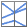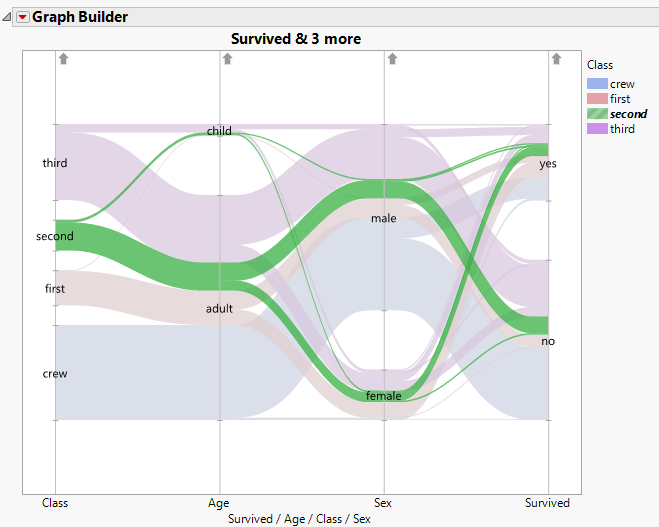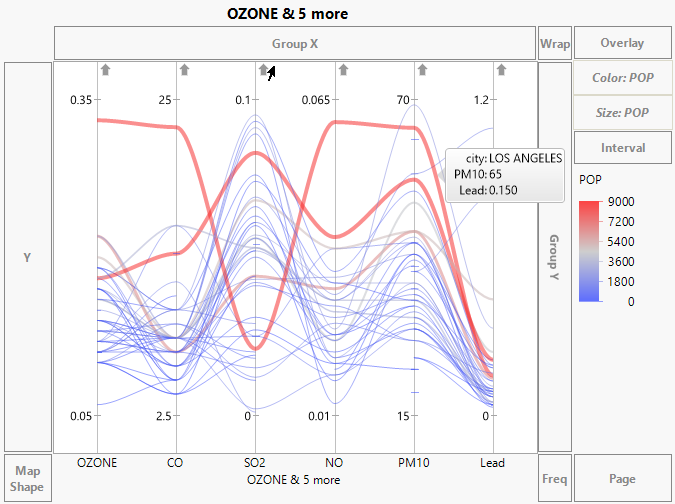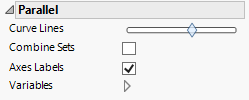Publication date: 05/24/2021

## Parallel

The Parallel elementconnects the values in a row across two or more variables. Drag two or more variables together to either the X or Y zone. The variable names appear as axis labels in the zone to which they were dragged.

Values for each continuous variable are plotted along lines (axes) parallel to the other axis.

Levels of categorical variables are represented by intervals on lines (axes) parallel to the other axis. The sizes of the intervals are proportional to the number of observations in each level of the categorical variable.

The values (for continuous variables) or bands (for categorical variables) are joined with continuous curves.

When all variables are categorical, there is a band for every combination of levels of the categorical variables. The bands split as they move from left to right. In the first interval corresponding to a categorical variable, there is a band for every level of that categorical variable. In the last interval corresponding to categorical variables, there is a band for every possible combination of the categorical variables.

Figure 3.42 Example of Categorical Bands Using Titanic.jmpIn Figure 3.42, the band containing all second-class passengers is selected. The parallel plot shows that most were adults, there were more males than females, and slightly fewer survived than did not survive.

The values or levels of the variables are connected with lines or curves using the Curve Lines option.

To color the curves by the values of a variable, drag the column of interest to the Color zone. The categorical or continuous color theme selected in your Preferences is shown in the Legend zone.

To size the curves by the values of a variable, drag the column of interest to the Size zone.

To change the direction of a variable’s axis, click the arrow at the top of the vertical line denoting the variable’s axis.

To move a variable and its axis, click and drag the axis.

Tip: Hover over a curve to see a label giving information about the corresponding row.

Figure 3.43 shows a parallel plot for six variables in the Cities.jmp data table. The variable POP is used both as a Color and Size variable. The curve for Los Angeles is labeled.

Figure 3.43 Parallel Plot for Pollution Data in Cities.jmpTips:

You can add a line element to a parallel plot to show a summary relationship across the parallel plot.

You can add reference lines for specification limits. For information, see Spec Limits in Using JMP.

#### Scaling

By default, the scales for the values of the variables are adjusted so that the minimum and maximum values are plotted at the same level. For example, in Figure 3.43, the values of each of the variables have an identical vertical spread. Each vertical line is labeled by the minimum and maximum values of the variables.

In Figure 3.43, the scales for CO and PM10 differ greatly from the scales of the other variables. When your variables are measured on very different scales, this scaling enables you to see differences clearly.

Figure 3.44 Parallel OptionsCurve Lines

Adjusts the amount of curvature of the curves that connect points. Place the slider all the way to the left for lines. As you move the slider to the right, the degree of curvature increases.

Combine Sets

(Applicable only if you have three or more categorical factors.) For categorical variables, when Combine Sets is not selected, bands split as they move from left to right. In the last interval that corresponds to a categorical variable, there is a band for every possible combination of the categorical variables. Selecting the Combine Sets option causes the bands not to split. Each interval that follows a categorical variable shows a band for each level of that variable.

Axes Labels

Removes the axis labels from the display.

Variables

Shows or hides graph elements for variables, or re-orders the display of variables.

Note: These options do not apply to variables in the Group X, Group Y, Wrap, or Page zones.

Check boxes are followed by the zone designation and the name of the variable. Use check boxes to do the following:

Show or hide the elements corresponding to a variable in a zone.

Add or remove the effect of applying the Color, Size, Shape, or Freq variable to the variable in the zone.

Tip: If you have multiple graphs, you can color or size each graph by different variables. Drag a second variable to the Color or Size zone, and drop it in a corner. In the Variables option, select the specific color or size variable to apply to each graph.

Use arrows to re-order the display if there are multiple variables in a zone. Highlight a variable name and click an arrow to reposition it.

For an example using Variables, see Example of an Area and Line Chart.